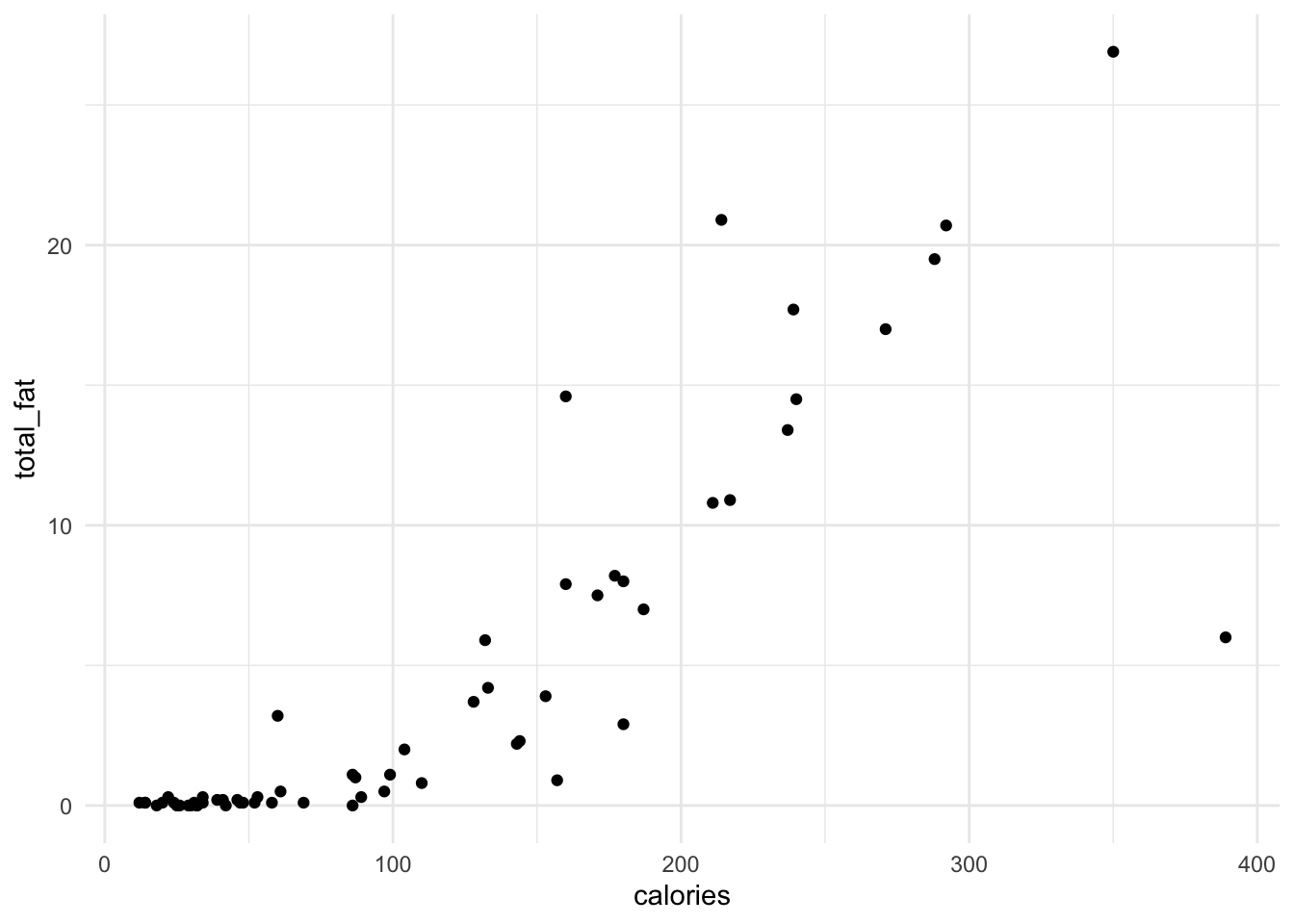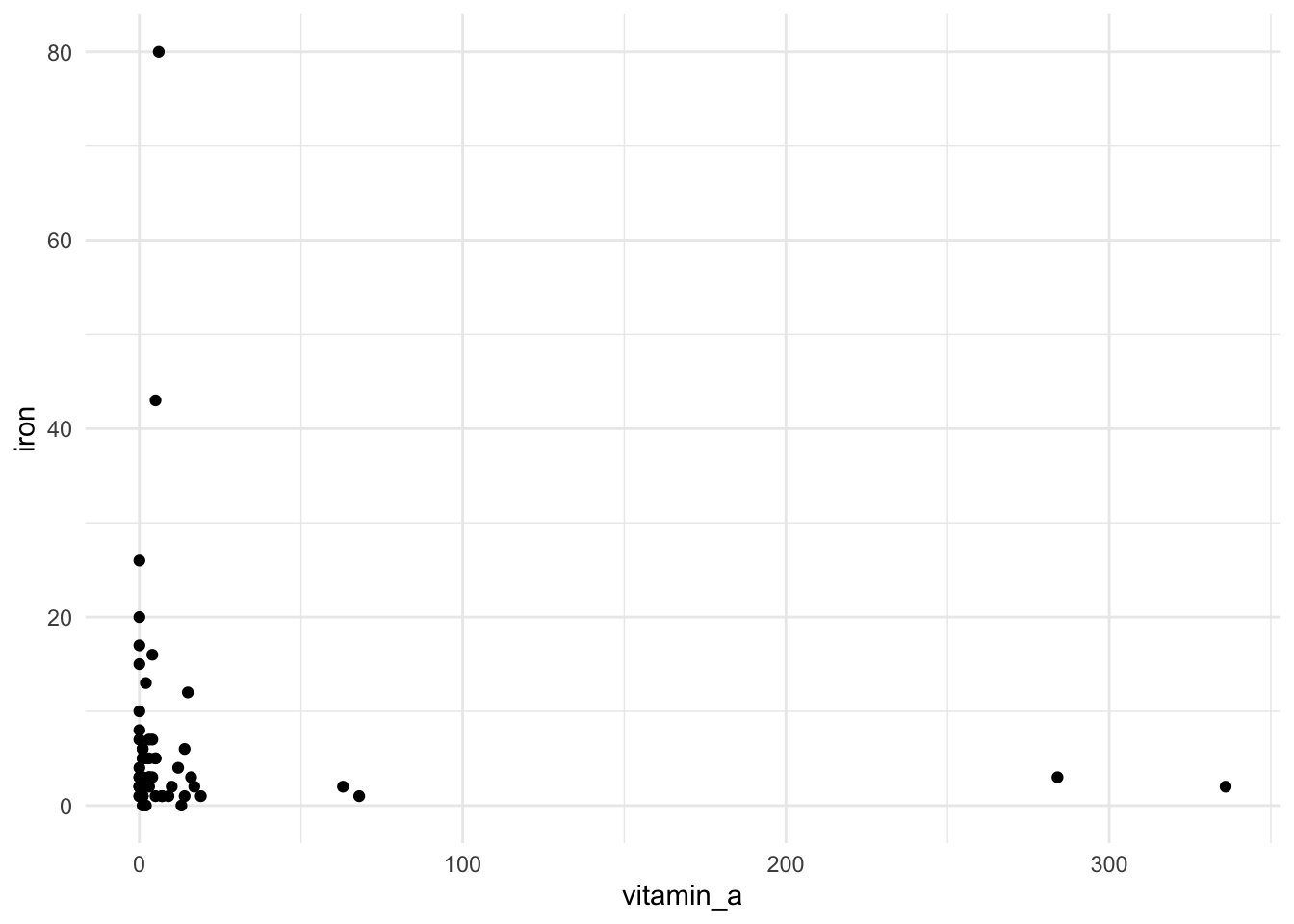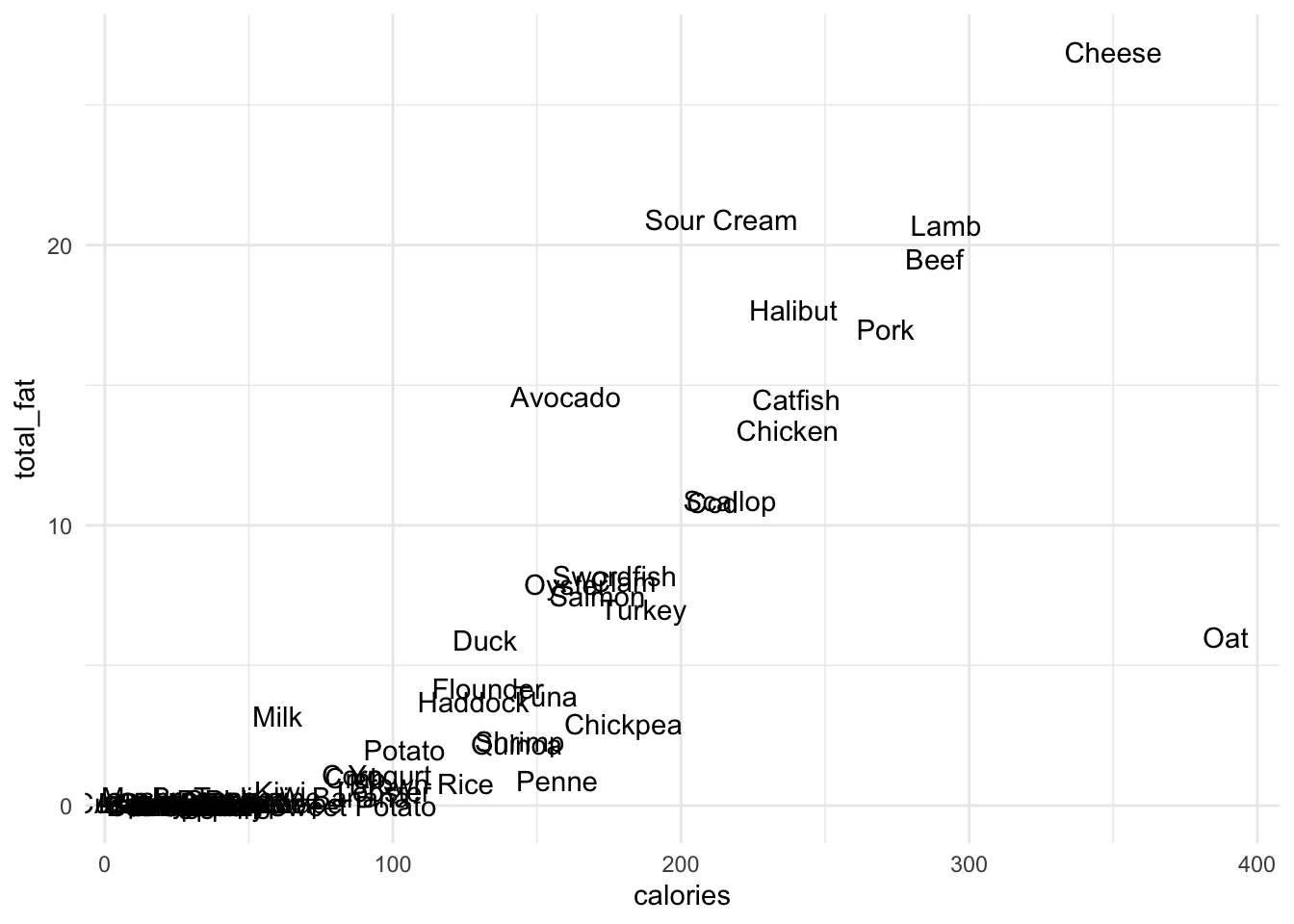## Getting Started

Before running this notebook, select “Session > Restart R and Clear Output” in the menu above to start a new R session. This will clear any old data sets and give us a blank slate to start with.

After starting a new session, run the following code chunk to load the libraries and data that we will be working with today.

I have set the options `include=FALSE` and `message=FALSE` to avoid cluttering the solutions with all the output from this code.

## Grammar of Graphics

### Elements

Data visualization is an incredibly important skill and a task that R is particularly well-designed for. We are going to learn and use the ggplot2 package for building beautiful and informative graphics. The package makes it easy to build fairly complex graphics in a way that is guided by a general theory of data visualization. The only downside is that, because it is built around a theoretical model rather than many one-off solutions for different tasks, it has a steep initial learning curve. These notes will, hopefully, make this as painless as possible.

The core idea of the grammar of graphics is that visualizations are composed of independent layers. To describe a specific layer, we need to specify several elements:

• data: the data set from which data will be taken to construct the plot
• geom: a description of what kinds of objects to plot (i.e., points, labels, or boxes)
• aes: a mapping from elements of the plot to columns in our data set (i.e., the position on the x-axis or the color of our points); it stands for aesthetics

You can describe virtually any type of visualization by putting together these elements.

To show how to use the grammar of graphics, we will start by using the food data set introduced in the previous notes, with each row describing a particular item of food along with various nutritional information. The first plot we will make is a scatter plot that investigates the relationship between calories and the total fat (in grams) that are in a 100g portion of each food item. In the language of the grammar of graphics we can describe this with the following elements:

• data: our data set is called `food`
• geom: we will build a plot with a points geometry; each row of data is represented by a point
• aes: the x-axis will be associated with `calories` and the y-axis with `total_fat`

### Scatter plot example

The easiest way to understand how we specify these elements within ggplot is by seeing an example. Here is the code to specify the data, geom, and aes:

``````food %>%
ggplot() +
geom_point(aes(x = calories, y = total_fat))``````In the first line we specify the data set (`food`), which is then piped (`%>%`) into the function `ggplot`, which instructs R to start a new plot. Next, we add (`+`) a layer to the plot. This layer uses a points geom (`geom_point`) and describes two aes values, `x = calories` and `y = total_fat`.

In order to make a similar plot with different variables, or a different data set, you can copy this code and change the associated variable names (`food`, `calories`, and `total_fat`). In the code below create another scatterplot from the food data set, choosing any two variables for the two axes:

``````food %>%
ggplot() +
geom_point(aes(x = vitamin_a, y = iron))``````In the next few classes we will see how to modify and build on this basic structure to create more complex graphics.

### Text Geometries

Let’s go through several other choices of geometries that we could have in the plot. There are many of these, but in general you can create most plots with only a small number of geometry types. To start, we will use the geometry `geom_text`, which puts a small label in place of the points in our previous example.

The text geometry needs an additional aes called `label` to describe what variable in the data set should be used as the label. Here, we use the variable called `item` to label each point with the name of the specific food item in question (the column is called `item`):

``````food %>%
ggplot() +
geom_text(aes(x = calories, y = total_fat, label = item))``````Can you now identify what food has the highest amount of fat? Or the highest calorie count? Hopefully!

You likely cannot, however, figure out what foods have the lowest amount of fat because the labels become too clumped together. In order to try to address this issue, we can use a slightly different geometry called `geom_text_repel`. It also places labels on the plot, but has logic that avoids intersecting labels. Instead, labels are moved away from the data points and connected (when needed) by a line segment:

``````food %>%
ggplot() +
geom_text_repel(aes(x = calories, y = total_fat, label = item))``````